### Statistical Inference and Lookup Degrees of Freedom

If you denote the ith measurement of the response byand the corresponding measurement of predictors by, then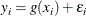where g is the regression function andare independent random errors with mean zero. If the errors are normally distributed with constant variance, then you can obtain confidence intervals for the predictions from PROC LOESS. You can also obtain confidence limits in the case whereis heteroscedastic but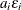has constant variance and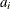are a priori weights that are specified using the WEIGHT statement of PROC LOESS. You can do inference in the case in which the error distribution is symmetric by using iterative reweighting. Formulas for doing statistical inference under the preceding conditions can be found in Cleveland and Grosse (1991) and Cleveland, Grosse, and Shyu (1992). Cleveland and Grosse (1991) show that standardized residuals for a loess model follow a t distribution withdegrees of freedom where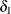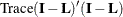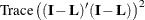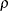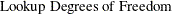The residual standard error that you find in the Fit Summary table is defined byThe determination ofis computationally expensive and is not done by default. It is computed if you specify the DFMETHOD=EXACT or DFMETHOD=APPROX option in the MODEL statement. It is also computed if you specify any of the options CLM, STD, and T in the MODEL statement. Note that the values of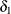,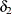, andare reported in the Fit Summary table.

If you specify the CLM option in the MODEL statement, confidence limits are added to the OutputStatistics table. By default, 95% limits are computed, but you can change this by using the ALPHA= option in the MODEL statement.